## Wednesday, January 31, 2018

### State of the Union

(Click on the comic if you can't see the full image.)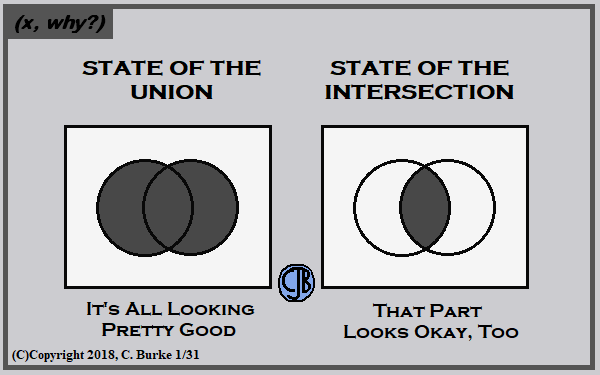Just don't start asking for complements!

Come back often for more funny math and geeky comics.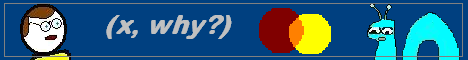## Sunday, January 28, 2018

### August 2017 Common Core Geometry Regents, Part 2

While I wait for the January 2018 Geometry Regents exams to become available, and for the go-ahead to publish the questions and answers, allow me to revisit the August 2017 exams, which I haven't gotten around to dealing with until now.

### August 2017, Geometry, Part II

Each correct answer is worth up to 2 credits. Work must be shown.

25. Sue believes that the two cylinders shown in the diagram below have equal volumes.

Is Sue correct? Explain why.

Answer: Sue is correct because the two cylinders have the same base area and the same height.
You didn't need to calculate the Volume to answer the question, but if you did, don't make any rounding mistakes!
V = (pi) (r)2 (h). In both cases, r = 5 and h = 11.5

26. In the diagram of rhombus PQRS below, the diagonals and intersect at point T, PR = 16, and QS = 30. Determine and state the perimeter of PQRS.

Answer: PR is perpendicular to QS, so the 4 triangles are congruent right triangles. The sides of the rhombus are the hypotenuses of the right triangles. PT = 16 / 2 = 8, QT = 30 / 2 = 15.
82 + 152 = PQ2
64 + 225 = PQ2
289 = PQ2
17 = PQ
Perimeter = 17 * 4 = 68

27. Quadrilateral MATH and its image M"A"T"H" are graphed on the set of axes below.

Describe a sequence of transformations that maps quadrilateral MATH onto quadrilateral M"A"T"H".

Answer: There a number of possible solutions.
One of them is a Rotation of 180 degrees about the axis followed by a Translation of +1, -1 (T+1,1).

Something like a reflection across y = -x or a dilation of scale factor -1 could also work in place of the rotation.

28. Using a compass and straightedge, construct a regular hexagon inscribed in circle O.

Answer: I can describe what you need to do, but showing the steps of a construction are always a problem for my blog.
Put the compass on a point on the circle. Draw an arc that goes through the center point, O, and draw an arc through the circle.
Go to the point on the circle you just found. Without changing the compass, make another arc on the circle. Repeat this until you go completely around the circle.
Your last arc should be through the point you started from.
Use the straightedge to connect the points on the circle. You have inscribed a hexagon in the circle.

The image below was taken from the state's answer key:

29. The coordinates of the endpoints of AB are A(2,3) and B(5,–1). Determine the length of A'B', the image of AB, after a dilation of 1/2 centered at the origin.

Answer: You do not need to graph the line segment or its image. The dilation with have a length of 1/2 the original.
Use the distance formula d = sqrt ( (5-2)2 + (-1-3)2 )
d = sqrt(9 + 16) = sqrt(25) = 5
length of AB is 5
Therefore, the length of A'B' is 2.5.

30. In the diagram below of triangle ABC and triangle XYZ, a sequence of rigid motions maps ∠A onto ∠X, ∠C onto ∠Z, and AC onto XZ.

Determine and state whether BC = YZ. Explain why.

Answer: Because ∠Amaps to ∠X and ∠C to ∠Z and AC to XZ, triangles ABC and XYZ are congruent by ASA. This means that BC is congruent to YZ because the corresponding parts of congruent triangles are congruent.
Additionally, rigid motions preserve side length and angle measure.

31. Determine and state the coordinates of the center and the length of the radius of a circle whose equation is x2 + y2 - 6x = 56 - 8y.

Answer: Standard form for a circle is (x -h)2 + (y - k)2 = r2. This equation needs to be rewritten and factored, by completing the square.

x2 + y2 - 6x = 56 - 8y.
x2 - 6x + y2 + 8y = 56
Half of -6 is -3, (-3)2 = 9, add 9 to both sides
Half of 8 is 4, (4)2 = 16, add 16 to both sides
x2 - 6x + 9 + y2 + 8y + 16 = 56 + 9 + 16
x2 - 6x + 9 + y2 + 8y + 16 = 56 + 9 + 16
(x - 3)2 + (y + 4)2 = 81, r2 = 81, so r = 9.
The center of the circle is (3, -4) and the radius is 9.

End of Part II

How did you do?

## Friday, January 26, 2018

### SCIENCE! With Scott: Imaginary Colors

(Click on the comic if you can't see the full image.)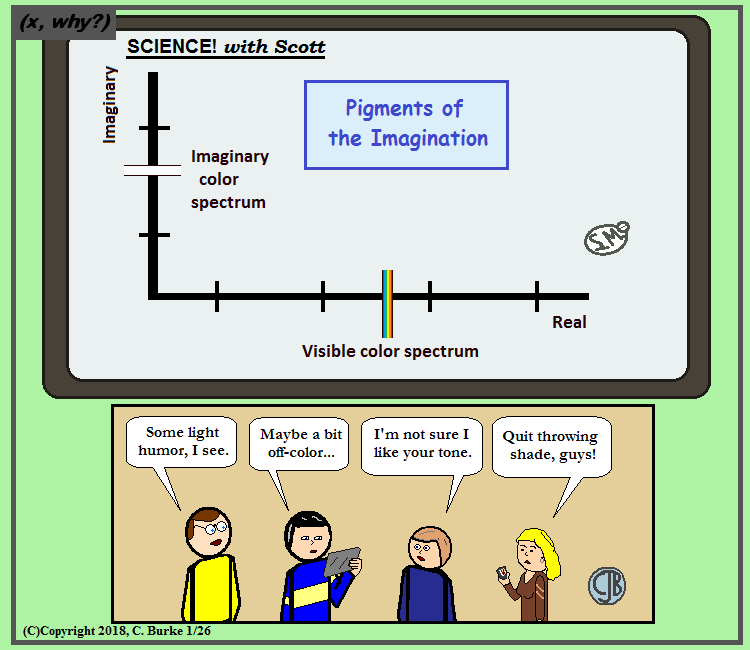Before a hue and cry of great intensity begins, humor flows along similar wavelengths so there's sometimes a saturation with with some puns.

Come back often for more funny math and geeky comics.## Wednesday, January 24, 2018

### Diamond Girl

(Click on the comic if you can't see the full image.)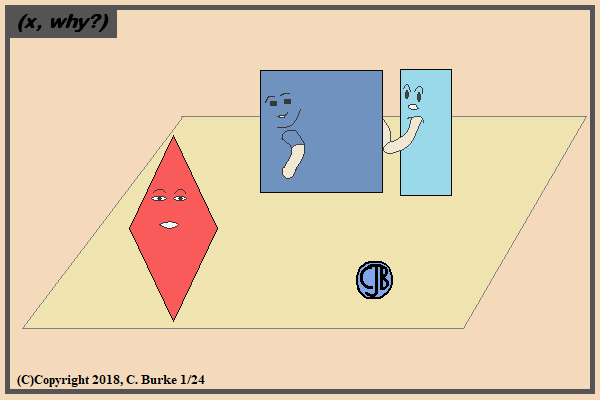The rectangle should tell the square to go fly a kite!

Don't do it. It's a trap-ezoid, for very loose definitions.

Come back often for more funny math and geeky comics.## Tuesday, January 23, 2018

### August 2017 Common Core Geometry Regents, Part 1

While I wait for the January 2018 Geometry Regents exams to become available, and for the go-ahead to publish the questions and answers, allow me to revisit the August 2017 exams, which I haven't gotten around to dealing with until now.

### August 2017, Geometry, Part I

1. A two-dimensional cross section is taken of a three-dimensional object. If this cross section is a triangle, what can not be the three-dimensional object?

(2) cylinder You can't take a cross section of a cylinder and get a triangle as a result. Vertical cross sections of cones and pyramids are triangles. Slicing off the corner of a rectangular prism will give you a triangle.

2. The image of triangle DEF is triangle D'E'F'. Under which transformation will the triangles not be congruent?

(4) a dilation with a scale factor of 3/2 centered at the origin A dilation will yield a similar triangle, but not a congruent one. However, choice (3) has a scale factor of 1, which won't change the size. (It won't do anything.)

3. The vertices of square RSTV have coordinates R(-1,5), S(-3,1), T(-7,3), and V(-5,7). What is the perimeter of RSTV?

(3) 4 (sqrt (20)) Use the distance formula to find the length of one side and then multiply by four, because it's a square with four equal sides.
d = sqrt( (-3 - -1)2 + (1 - 5)2)
= sqrt ( (-2)2 + (-4)2)
= sqrt ( 4 + 16 ) = sqrt (20), the length of ONE side of the square.

4. In the diagram below of circle O, chord CD is parallel to diameter AOB and mCD = 130.

What is mAC?

(1) 25
The semicircle ACDB is 180 degrees. Arc CD is 130. 180 - 130 = 50
The sum of arcs AC and DB = 50, but because the chord is parallel to the diameter, the two arcs are equal.
Therefore arc AC = 50 / 2 = 25.

5. Iin the diagram below, AD intersects BE at C, and AB || DE.

If CD = 6.6 cm, D# = 3.4 cm, CE = 4.2 cm, and BC = 5.25 cm, what is the length of AC, to the nearest hundredth of a centimeter?

(4) 8.25
Because of the vertical angles and the alternate interior angles, we know that the triangles are similar. That means that the corresponding sides are proportional.
CD / CE = AC / BC
6.6 / 4.2 = AC / 5.25
4.2 AC = (6.6)(5.25)
AC = (6.6)(5.25)/4.2 = 8.25

6. As shown in the graph below, the quadrilateral is a rectangle.

Which transformation would not map the rectangle onto itself?

(3) a rotation of 180° about the origin
Rotating 180 degrees about the origin would put the rectangle in quadrants II and III.
Rotating 180 degrees about the point (4, 0) would map it onto itself because (4, 0) is the center of the rectangle.

7. In the diagram below, triangle ACD has points B and E on sides AC and AD, respectively, such that DE||CD, AB = 1, BC = 3.5, and AD 18.

What is the length of AE, to the nearest tenth?

(4) 4.0
Since the lines are parallel, triangles ABE and ACD are similar and their sides are proportional.
AB / AC = AE / AD
1 / 4.5 = AE / 18
4.5 AE = 18
AE = 4.0

8. In the diagram below of parallelogram ROCK, m∠C is 70° and m∠ROS is 65°.

What is m∠KSO?

(4) 135°
Angle R is congruent to Angle C, so angle R is 70.
Angle KSO is the exterior angle for triangle ROS. The remote angles are 65 and 70, so 65 + 70 = 135.

9. In the diagram below, ∠GRS ∠ART, GR 36, SR 45, AR 15, and RT 18.

Which triangle similarity statement is correct?

(4) Triangle GRS is not similar to triangle ART.
45 * 15 = 675
36 * 18 = 648
The sides are not proportional (which should've obvious because 5*5=25 and 6*8=48).

10. The line represented by the equation 4y = 3x + 7 is transformed by a dilation centered at the origin. Which linear equation could represent its image?

(1) 3x - 4y = 9
A dilation would not change the slope. The slope of the original line is 3/4.
Choice (1) has a slope of 3/4.

11. Given triangle ABC with m∠B = 62° and side AC extended to D, as shown below.

Which value of x makes AB = CB?

(4) 121°
For AB to be congruent to CB, angle A must be congruent to angle ACB.
So y + y + 62 = 180
2y + 62 = 180
2y = 118
y = 59
Using the Remote Angle Theorem, x = 62 + 59 = 121.

12. In the diagram shown below, PA is tangent to circle T at A, and secant BC is drawn where point B is on circle T.

If PB = 3 and BC = 15, what is the length of PA?

(2) 3 * SQRT(6)
(PA)2 = (PB)(PC) = (3)(3+15) = 54
PA = SQRT(54) = SQRT(9)*SQRT(6) = 3*SQRT(6)

13. A rectangle whose length and width are 10 and 6, respectively, is shown below. The rectangle is continuously rotated around a straight line to form an object whose volume is 150π.
Which line could the rectangle be rotated around?

(3) the vertical line of symmetry
Which line is used will determine what the radius is and what the height is.
Quick check: if the height is 10, then r2 = 150 / 10 = 15, which isn't a perfect square.
if the height is 6, then r2 = 150 / 6 = 25, which is a perfect square, and the radius is 5.
Since the long side has a length of 10, then a radius of 5 means you need to use the vertical line of symmetry and not the short side.

14. If ABCD is a parallelogram, which statement would prove that ABCD is a rhombus?

(3) AC is perpendicular to BD
What makes a parallel also a rhombus is that the consecutive sides are congruent. This isn't given. Choices (1) and (2) are also true for parallelograms that aren't rhombuses. (Rhombi?) However, another property of rhombuses is that the diagonals are perpendicular, which is not true for other parallelograms.

15. To build a handicapped-access ramp, the building code states that for every 1 inch of vertical rise in height, the ramp must extend out 12 inches horizontally, as shown in the diagram below.

What is the angle of inclination, x, of this ramp, to the nearest hundredth of a degree?

(1) 4.76
tan x = opp / adj
tan x = 1 / 12
x = tan-1(1/12) = 4.763...

More to come. Check back later ...

Continuing...

16. In the diagram below of triangle ABC, D, E, and F are the midpoints of AB, BC, and CA, respectively.

What is the ratio of the area of triangle CFE to the area of triangle CAB?

(4) 1:4
Because D, E, and F are midpoints, the DE, EF, and FD are all midsegments, which are half the length of the side of CAB that they are parallel to. This is enough information to show that the four smaller triangles are all congruent, which makes each one 1/4 of the total area. Thus, 1:4.

17. The coordinates of the endpoints of are A(-8,-2) and B(16,6). Point P is on AB. What are the coordinates of point P, such that AP:PB is 3:5?

((1) (1,1)
3 + 5 = 8, so point P is 3/8 of the distance from A to B.
change in x-coordinate: (16 - (-8)) * (3/8) = 24 * (3/8) = 9
change in y-coordinate: (6 - (-2)) * (3/8) = 8 * (3/8) = 3
P is at (-8 + 9, -2 + 3) = (1, 1).

18. Kirstie is testing values that would make triangle KLM a right triangle when LN is an altitude, and KM = 16, as shown below.

Which lengths would make triangle KLM a right triangle?

(2)LM = 12 and NM = 9
Working through each of the choices:
Choice (1), LM = 13, KN = 6. This means that MN = 16 - 6 = 10 and LN = sqrt((6)(10)) = sqrt(60).
Also LN = sqrt((13)2 - (10)2) = sqrt(169 - 100) = sqrt(69). Contradiction.
Choice (2), LM = 12 and NM = 9. This means KN = 7 and LN = sqrt ((9)(7)) = sqrt(63).
Also LN = sqrt((12)2 - (9)2) = sqrt(144 - 81) = sqrt(63). This is the answer.
Choice (3), KL = 11 and KN - 7. This means MN = 9 and LN = sqrt ((9)(7)) = sqrt(63).
Also LN = sqrt((11)2 - (7)2) = sqrt(121 - 49) = sqrt(72). Contradiction.
Choice (4), LN = 8 and NM = 10. This means KN = 6 and LN = sqrt ((10)(6)) = sqrt(60). Contradiction.

19. In right triangle ABC, m∠A = 32°, m∠B = 90°, and AC = 6.2 cm. What is the length of BC, to the nearest tenth of a centimeter?

(1) 3.3
sin 32° = BC/AC
.5299 = BC / 6.2
BC = 3.285, approx 3.3

20. The 2010 U.S. Census populations and population densities are shown in the table below.

Based on the table above, which list has the states’ areas, in square miles, in order from largest to smallest?

(1) Illinois, Florida, New York, Pennsylvania
Divide population by density to get each area
Forida: 18,801,310 / 350.6 = 53626 sq mi
Illinois: 12,830,632 / 231.1 = 55520 sq mi
New York: 19,378,102 / 411.2 = 47126 sq mie
Pennsylvania: 12,702,379 / 283.9 = 44742 sq mi

21. In a right triangle, sin (40 - x)° = cos (3x)°. What is the value of x?

(4) 25
sin (O) = cos (90 - O)
So 40 - x = 90 - 3x
and 2x = 50
Therefore x = 25

22. A regular decagon is rotated n degrees about its center, carrying the decagon onto itself. The value of n could be

(4) 252°
A decagon has 10 sides, so a rotation of (360 / 10) = 36 degrees would rotate the figure onto itself, as would any multiple of 36 degrees. Divide each choice by 36 to see which works. (Number Sense would tell you only (4) is possible)

23. In a circle with a diameter of 32, the area of a sector is 512π/3. The measure of the angle of the sector, in radians, is

(2) 4π/3
The radius of the circle is 16.
The area of a sector of a circle is (1/2)r2
(1/2)(16)2 ∅ = 512π/3
128 ∅ = 512π/3
∅ = 4π/3

If you forgot this formula, the area of the entire circle is 256π
(512π/3) / 256π = 2/3
2/3 of a circle = 2/3 * (2π) = 4π/3

24. What is an equation of the perpendicular bisector of the line segment shown in the diagram below?

(4) 2y - x = 0
The line has a slope of -2, so the perpendicular slope is 1/2.
The midpoint of the line is (0, 0), which gives you the y-interept.
So y = 1/2 x + 0
y - 1/2 x = 0, or
2y - x = 0.

End of Part I

### August 2017 Common Core Algebra 1 Regents, Part 4

Continuing with the August 2017 Algebra 1 Regents.
Here are the Part I questions, answers, and explanations.
Here are the Part II questions, answers, and explanations.
Here are the Part III questions, answers, and explanations.

### August 2017, Algebra 1 (Common Core), Part IV

A correct answer will receive 6 credits. For all questions in this part, a correct numerical answer with no work shown will receive only 1 credit.

37. Zeke and six of his friends are going to a baseball game. Their combined money totals \$28.50. At the game, hot dogs cost \$1.25 each, hamburgers cost \$2.50 each, and sodas cost \$0.50 each. Each person buys one soda. They spend all \$28.50 on food and soda.
Write an equation that can determine the number of hot dogs, x, and hamburgers, y, Zeke and his friends can buy.

Graph your equation on the grid below.
Determine how many different combinations, including those combinations containing zero, of hot dogs and hamburgers Zeke and his friends can buy, spending all \$28.50. Explain your answer.

Answer: There are 7 people. Each buys a soda, which are \$0.50 each. .50 * 7 = \$3.50.
\$28.50 - 3.50 = 25.00 to spend on hot dogs and hamburgers.

The equation is 1.25x + 2.50y + 3.50 = 28.50 or 1.25x + 2.50y = 25.00
The price of a hot dog times the number of hot dogs plus the price of a hamburger times the number of hamburgers plus the price of the sodas equals \$28.50. Note that the question states that all the money was spent and that you are looking for an equation, not an inequality.

The number of \$1.25 hot dogs that can be bought for \$25.00 is 20. The number of \$2.50 hamburgers that can be bought is 10.
There will be 11 possible combinations, which appear on the graph below. You don't need to list them, but they are:
(20, 0), (18, 1), (16, 2), (14, 3), (12, 4), (10, 5), (8, 6), (6, 7), (4, 8), (2, 9), (0, 10).

Here is the graph:

End of Part IV
End of Exam

How did you do?

Reminder: I've been asked not to post the questions to newer exams for at least a week after it.
So I probably won't be able to write up the answers to today's tests until after February 1.

## Monday, January 22, 2018

### Hot Dogs, Buns and Laundry

(Click on the comic if you can't see the full image.)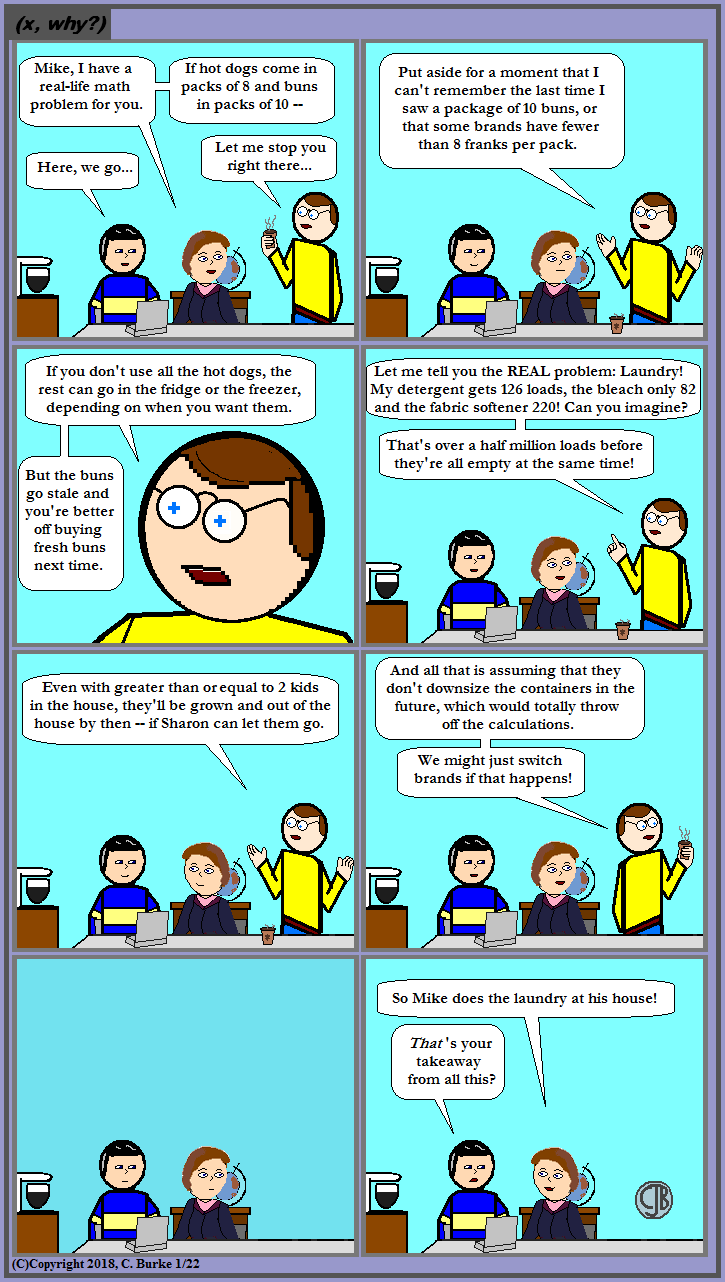Household Math 101: Division of Chores

Don't ask a math person a question if you don't want to know the answer.

For anyone wondering, Mike was referring to non-chlorine bleach but try fitter all that, without resorting to brand names!

I've also discovered that I dislike making close-up versions of my characters even more than when I simply "zoom in" on their pixelated images.

Come back often for more funny math and geeky comics.## Thursday, January 18, 2018

### August 2017 Common Core Algebra 1 Regents, Part 3

Continuing with the August 2017 Algebra 1 Regents.
Here are the Part I questions, answers, and explanations.
Here are the Part II questions, answers, and explanations.

### August 2017, Algebra 1 (Common Core), Part III

Each correct answer will receive 4 credits. For all questions in this part, a correct numerical answer with no work shown will receive only 1 credit.

33. Loretta and her family are going on vacation. Their destination is 610 miles from their home. Loretta is going to share some of the driving with her dad. Her average speed while driving is 55 mph and her dad’s average speed while driving is 65 mph.

The plan is for Loretta to drive for the first 4 hours of the trip and her dad to drive for the remainder of the trip. Determine the number of hours it will take her family to reach their destination.

After Loretta has been driving for 2 hours, she gets tired and asks her dad to take over. Determine, to the nearest tenth of an hour, how much time the family will save by having Loretta’s dad drive for the remainder of the trip.

Answer: Loretta drives 55 mph for 4 hours. Distance = rate X time, d = rt
Loretta drives d = (55)(4) = 220 miles of the 610 miles of the trip.
Her Dad will drive the remaining 610 - 220 = 390 miles
Using d = rt again, 390 = (65)t
t = 6 hours her Dad drives.

Note: you will neither gain nor lose a point whether or not you capitalize the "d" in "Dad", but I'm a "Dad", so there. But I digress.

The total number of hours it should take it 4 + 6 = 10 hours of total driving time.

Second part: If Loretta only drives 2 hours, then they have only traveled 55 * 2 = 110 miles.
Dad has to drive 610 - 110 = 500 miles
500 / 65 = 7.69... or 7.7 hours
To the nearest tenth, the family will drive for 2 + 7.7 = 9.7 hours.
The family will save 0.3 hours in this situation.

34. The heights, in feet, of former New York Knicks basketball players are listed below

Using the heights given, complete the frequency table below
Based on the frequency table created, draw and label a frequency histogram on the grid below.
Determine and state which interval contains the upper quartile. Justify your response

Answer: The frequency table will look like the following. Note that you could have just the tally marks or just the numerical value, and you would receive credit for this portion of the question.

Note that they wanted a frequency histogram, not a bar graph, line graph or anything cumulative. It's important to leave a blank column between 0 and the first bar to indicate the gap, and you should include the little squiggle (which they are inconsistent about demanding).
Remember to add title, labels, scale, etc. Also, do not shade or draw anything to represent 0 for 6.6-6.7.

You can find the upper quartile by either writing out the numbers in order, then finding the median and quartiles. (There are 29 numbers, the 15th is the median, and the upper quartile is between the 7th and 8th numbers of the top half of data. This will give you 6.5.) Otherwise, you can say that 29 divided by 4 is 7.25, and if you could back from the last tally mark on the frequency table, you will find that the 7th and 8th marks are both in the interval 6.4-6.5.

35. Solve the following system of inequalities graphically on the grid below and label the solution S.

3x + 4y > 20 x < 3y - 18

Answer: Remember to label the axis. The inequalities used are greater than and less than, so neither will have a solid line.
If you are using the graphing calculator to help with the graph, you need to rewrite the equations is slope-intercept form.

 3x + 4y > 20 4y > -3x + 20 y > -3/4 x + 5 x < 3y - 18 x + 18 < 3y (1/3)x + 6 < y y > (1/3)x + 6

(3, 7) is NOT a solution because it is on the broken line, and the line is not part of the solution.

36. An Air Force pilot is flying at a cruising altitude of 9000 feet and is forced to eject from her aircraft.
The function h(t) = -16t2 + 128t + 9000 models the height, in feet, of the pilot above the ground, where t is the time, in seconds, after she is ejected from the aircraft.

Determine and state the vertex of h(t). Explain what the second coordinate of the vertex represents in the context of the problem.

After the pilot is ejected, what is the maximum number of feet she was above the aircraft's cruising altitude? Justify your answer.

Answer: The vertex can be found from the axis of symmetry, which is x = (-b) / (2a), where a = -16 and b = 128.
x = -(128) / (2(-16)) = -128 / -32 = 4
y = h(4) = -16(4)^2 + 128(4) + 9000 = 9256
Oddly, in this particular problem, no actual work was needed, and if you found the answer in your calculator by graphing and finding the maximum, you would have been fine. I do not recommend that approach, except to check your math.

The coordinates of the vertex are (4, 9256) where the second coordinate is the height of the pilot above ground level in feet.

The maximum number of feet the pilot will rise above the plane is 9256 - 9000 = 256 feet.

## Wednesday, January 17, 2018

### (x, why?) Mini: Noah

(Click on the comic if you can't see the full image.)He built it in degrees.

Come back often for more funny math and geeky comics.## Tuesday, January 16, 2018

### Center

(Click on the comic if you can't see the full image.)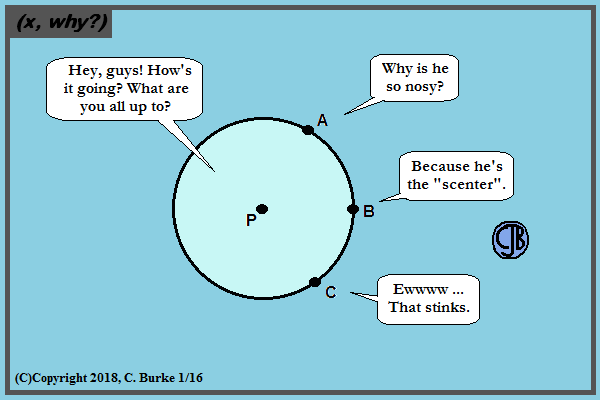Maybe it should be point PU?

Come back often for more funny math and geeky comics.## Friday, January 12, 2018

### Nonplussed

(Click on the comic if you can't see the full image.)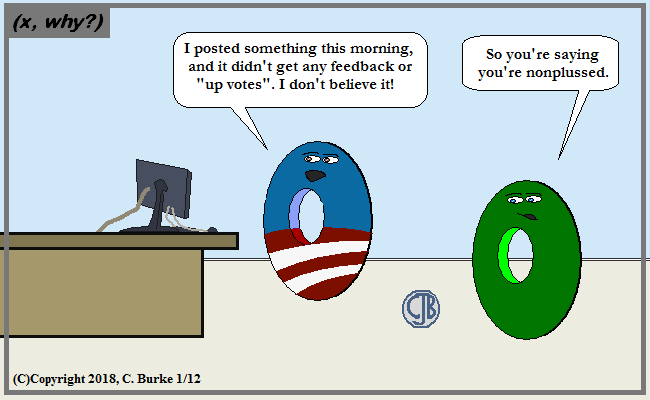At least there was nothing negative.

Come back often for more funny math and geeky comics.### August 2017 Common Core Algebra 1 Regents, Part 2

I recently realized that students are reviewing the August 2017 Algebra 1 Regents, and I never wrote up the open-ended problems.
Here are the Part I questions, answers, and explanations.

### August 2017, Algebra 1 (Common Core), Part II

25. A teacher wrote the following set of numbers on the board:

Explain why a + b is irrational, but b + c is rational.

Answer: The sum a + b is irrational because the sum of an irrational number and a rational number is always irrational.
The sum of b + c is rational because the square root of 225 is 15, which is a rational number. The sum of two rational numbers is always rational.

26. Determine and state whether the sequence 1, 3, 9, 27,… displays exponential behavior. Explain. how you arrived at your decision.

Answer: The sequence displays exponential behavior because it has a common ratio.
3 / 1 = 3
9 / 3 = 3
27 / 9 = 3

27. Using the formula for the volume of a cone, express r in terms of V, h, and π. how you arrived at your decision.

V = 1/3 π r 2 h
3 V = π r 2 h
3 V / (πh) = r 2
square root of (3 V / (πh)) = r

28. The graph below models the cost of renting video games with a membership in Plan A and Plan B.

Explain why Plan B is the better choice for Dylan if he only has \$50 to spend on video games, including a membership fee.

Bobby wants to spend \$65 on video games, including a membership fee. Which plan should he choose? Explain your answer.

Answer: According to the graph, Bobby can get 14 games under Plan B but he can only get 12 games under Plan A.
According to the graph, if Bobby plans to spend \$65, then both plans will give him the same number of games because that is the point where the two plans are the same.

29. Samantha purchases a package of sugar cookies. The nutrition label states that each serving size of 3 cookies contains 160 Calories. Samantha creates the graph below showing the number of cookies eaten and the number of Calories consumed.

Explain why it is appropriate for Samantha to draw a line through the points on the graph.

Answer: Samantha should draw a line through the points on the graph because she could eat only 1 or 2 cookies, or even a part of a cookie.

30. A two-inch-long grasshopper can jump a horizontal distance of 40 inches. An athlete, who is five feet nine, wants to cover a distance of one mile by jumping. If this person could jump at the same ratio of body-length to jump-length as the grasshopper, determine, to the nearest jump, how many jumps it would take this athlete to jump one mile.

Commentary: I'm surprised that they didn't include the unit conversions necessary to complete this problem. However, they are in the back of the test book.

Answer: The grasshopper is 2 inches and can jump 40 inches, which is a ratio of 40:2, or 20 times his body length.
The person is 5'9", which converts to 5.75 feet (because 9/12 of a foot is .75 feet). Multiply this by 20: 5.75 * 20 = 115 feet per jump.
One mile is 5280 feet, so 5280 / 115 = 45.9, which rounds to 46 jumps would be needed.

If you got as far as finding 115 feet (or 1380 inches), you would have gotten one point. You need to get to 46 jumps, rounded correctly, to get the other.

31. Write the expression 5x + 4x2(2x + 7) - 6x2 - 9x as a polynomial in standard form.

Answer: Use the Distributive Property, and then Combine Like Terms
Standard form means the term with the highest exponent goes first and then in decreasing order.
Remember that the sign goes with the term after it, and the first term here is positive (+).

5x + 4x2(2x + 7) - 6x2 - 9x
5x + 8x3 + 28x2 - 6x2 - 9x
8x3 + 28x2 - 6x2 + 5x - 9x
8x3 + 22x2 - 4x

32. Solve the equation x2 - 6x = 15 by completing the square

Answer: b = -6, so b / 2 = -3, which means that (x - 3) will be in the answer.
Also, (b / 2)2 = 9. Add 9 to both sides to begin.

x2 - 6x + 9 = 15 + 9
(x - 3)2 = 24
x - 3 = +(24)(1/2)
x = 3 +(24)(1/2)

It isn't necessary to reduce (radical (24)) into (2 X radical (6)).

End of Part II.
How did you do?

## Thursday, January 11, 2018

### The Roman One

(Click on the comic if you can't see the full image.)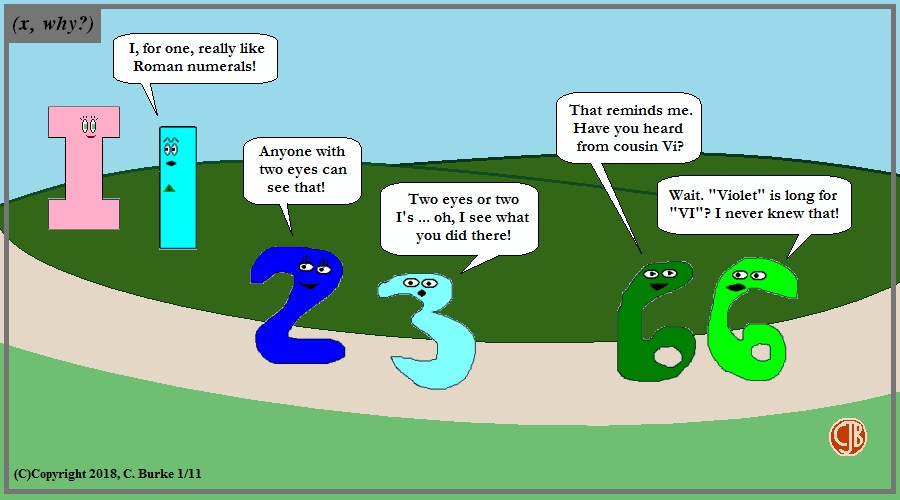100 what 1 did there?

Come back often for more funny math and geeky comics.## Monday, January 08, 2018

### New Mersenne Prime

(Click on the comic if you can't see the full image.)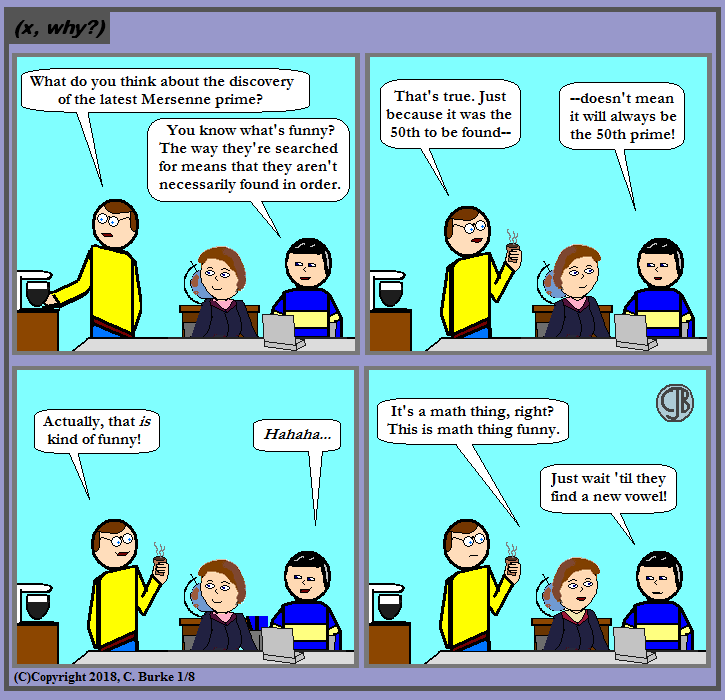Joke's on Ken. You can buy a vowel!

First, here's a previous comic from 2008 about Mersenne primes. They've found a few more since then.

For those who don't know, Mersenne primes, they are a special subset of primes that could be written in the form of 2prime# - 1.

I'd say I'm waiting for the speculation about 2Mersenneprime# - 1, but I wouldn't be surprised if that's already been done.

Come back often for more funny math and geeky comics.## Thursday, January 04, 2018

### Problem of the Day: Quadratic and Absolute Value Functions

During the break, an online friend, who knows I'm a math teacher (the user handle @mrburkemath is generally a tip-off), sent me the following math review problem:

"Given the functions h(x) = |x - 4| + 1 and k(x) = x2 + 3, which intervals contain a value of x for which h(x) = k(x)?
This was followed by a list of intervals in the form number < x < number. I've left these out as I have to assume the entire problem is copyrighted from a review book. I'm hoping my excerpt is covered by "fair use".

First thing I said what, in a purely multiple-choice format, just plug the two functions into a calculator (or use an online app, if you're at home without one) and look for the answers. Then select the intervals that include those values.

Otherwise, we can work it out. First thing to realize in that dealing with quadratics and absolute values, there can be, at most, two real number answers. (The problem didn't involve imaginary roots.)

The friend wasn't sure if she was supposed to set each equal to zero and solve, or set them equal to each other. It's in the question: h(x) = k(x).

So here is what we have:

h(x) = k(x)
|x - 4| + 1 = x2 + 3

First thing is to isolate the absolute value, by subtracting 1 from each side:

|x - 4| = x2 + 2

Many of you can skip the mini-review I'm going to do right now.
When solving an absolute value equation like |x - 4| = 7, you have to split the equation into two possibilities, one positive, one negative, and solve each.

 x - 4 = 7 x - 4 = -7

Applying that rule to this equation, you get
When solving an absolute value equation like |x - 4| = 7, you have to split the equation into two possibilities, one positive, one negative, and solve each.

 x - 4 = x2 + 2 x - 4 = -(x2 + 2) x - 4 = x2 + 2 x - 4 = -x2 - 2 0 = x2 - x + 6 x2 + x - 2 = 0 No real roots Factor (x + 2)(x - 1) = 0 x = -2 or x = 1

If you check the discriminant (b2 - 4ac) for the first equation, you will get a number less than 0, meaning that there are no real roots.

Going back to the original problem, they wanted you to select any of the intervals that contained either -2 or 1 or both.

Had they asked for the points of intersection, the values of the functions for those x values, then you have to plug them in:
h(-2) = |-2 - 4| + 1 = |-6| + 1 = 6 + 1 = 7, (-2, 7)
h(1) = |1 - 4| + 1 = |-3| + 1 = 3 + 1 = 4, (1, 4)

Simple, right?

## Monday, January 01, 2018

### 2017: A Teaching Year in Review

There's an adage that annoys teachers: Those who can, do; those who can't, teach. And yet we will also ponder the fate of those who "can't teach" -- often they become "consultants" after a year and a half in the classroom, becoming self-appointed experts at telling the rest of us how to do it.

In my case, it's not a matter of "can't teach" as much as "won't let me". Now, I'm not assigning blame, for a couple of reasons: first, I don't wish to burn bridges, nor kill my own career; second, I'm not entirely blameless in the situation. Stuff happens.

Looking back, 2017 started as a year of promises, and in five and a half months, many planted seeds seemed to be taking root and flowering. Bearing fruit, if you will. And then someone razed the garden.

In December 2017, after a few months in the ATR (Absentee Teacher Reserve) Pool, subbing for a few different schools, I received an inquiry about a math position in Park Slope. My previous school year had been dreadful, resulting in a "Developing" rating and the loss of nearly 20 pounds. I had been happy to be in the pool for those months, subbing for other teachers, occasionally pushing into and assisting in math classes, and generally covering whatever needed covering. As restful as this was, however, I couldn't see this being a career path for me, so I looked into the school that had contacted me.

There was several pluses that stuck out: it was in my old neighborhood, which was easy enough to get to; it was a small school inside a big building, shared with other small schools; and I had previously worked in the building, so I knew that there wasn't a history of "trouble" in the halls and stairwells.

The principal and AP were nice people, answered my questions as well as they could. Note that my first question, considering previous experiences, was "Is this position provisional or permanent?" Was I being hired to teach math at the school or to fill a void until the end of the year?

Honestly, I couldn't tell you at the time which answer I expected or even which I would've preferred, so long as I had an answer and knew what to expect. I was told that he had been told by "downtown" that whomever he hired that's who he hired. That was it.

So in January 2017, I was making progress getting to know my new Algebra and Geometry students, just as they were making progress. I found strengths in some low-performing students. A few others were suddenly "turned on" to math, which they hadn't been in middle school. And I made important connections with a couple of students who seemed troubled in their own ways. I found ways to reach them, to be able to talk to them (somewhat). They knew they could talk to me if anything was troubling them, disrupting their class time, distracting them from learning.

I made enough progress that I was once able to say to a reluctant talker, "I'm sorry but I only have a minute, so can we jump to the point where you start speaking to me?"

It was abrupt, but she opened up right away and asked what she had to, and I was able to answer her. Next time, I let her go back to her natural process before speaking, but I noted she spoke up a little sooner.

By spring, we could practically have a conversation without prompting. By June, she told me that she saw me as a kind of "mentor" figure. That same day I was called into the office for a meeting. I thought it was a post-observation conference or the end-of-year review (a little early). When I saw the ELL teacher in the room, I wondered if there had been an issue with one of my students.

No, the ELL teacher was also the union rep. She was there because I was being excessed. Let go. From a "permanent" position. I chose my words carefully because I didn't want to end my career. And I might have. I felt betrayed. And I felt I was betraying my students who had made connections with me and whom I thought I'd see again the following school year.

In the principal's mind, the position hadn't been filled yet. He was still searching, not that he'd ever mentioned this in the prior six months. Any protest or argument from me was cut off with a simple question: would I like to still be considered for the position?

As much as I wanted to tell him to shove it -- OF COURSE, I WANTED TO BE CONSIDERED FOR THE POSITION. I thought it was MY position. I was already making plans for next year, what to change in the curriculum, how to approach Geometry with my current Algebra students, how I wanted to redo the classroom. That ended it. Except I knew I wasn't going to be considered or we wouldn't be having the conversation. I'm too expensive, and new teachers are easy on the budget.

So I started making my good-byes to some of the students. I hope they do well. A couple of them were following me on social media and still contacted me early in the fall semester, but the contact has fallen off. I hope they found new mentors, ones that aren't going anywhere.

This would be a good point for a musical interlude, in place of my summer break, so I can stop rambling and recollect my thoughts.

The end of the summer brought another plot twist: the city and the union agreed to change the rules about placement. If I didn't find a position, if a school did not hire me, then there was a good chance that the city would select an open position somewhere in Brooklyn (possibly beyond) and place me there anyway, whether or not I wished to go there and without regard to the school's desires or ability to fit me in their budget. And that placement would be permanent unless -- here's the catch -- the teacher received a developing or unsatisfactory rating!

So what did this mean for me as a teacher: I could find myself in a horrible school, in a horrible neighborhood, in a horrible situation like the one that caused me to drop to a weight beginning with the number 1 (which I hadn't seen since the year started with the number 1). I might not be able to get out of it, if I couldn't find an open position. Moreover, if the school didn't want me there, then they could make my life miserable so I'd get an unsatisfactory rating!

Granted, it was no picnic for the schools, either, and it was no surprise that I got a few inquiries after this announcement, while at the same time, open positions started disappearing. I applied to quite a few places, but, sorry, I wasn't going back to middle school, and I wasn't traveling to the Bronx. (Note: it's a minimum two-hour travel, one-way, by subway to get to the Bronx from my house.)

Making my search more desperate, an email arrived stating that my first temporary assignment was at Cobble Hill High School for American Studies. Nice neighborhood, not a lot of teenagers in it. I spent one single week at that school and I rated it as probably the worst week of my teaching career. That week was an absolute disaster, and I didn't want to repeat it. I only name the school so I can give credit where it's due. I had maybe 1 or 2 difficult assignments during my eight weeks there in September and October. While the school still has its share of problems, I didn't encounter anything like the last time. And I'll give a shout-out to Stephanie (I hope I spelled it right), for taking good care of me and the other ATRs assigned there. On my last day there, I told her that I wish I could take her with me.

Okay, so what about that forced assignment? It might still be coming for all I know. We keep hearing that math is a shortage area. And there are openings, but schools are still playing games.

In mid-September, a former colleague, now an assistant principal reached out to me through the DOE email and through Facebook (we're not "friends" on Facebook) to let me know about an opening in Queens. I wasn't thrilled with the idea of Queens, but with the future uncertain, it paid to check it out. Basically, it was a temporary position for someone who might be coming back soon, might be filing an extension, or might be retiring (after exhausting extensions). I met with the principal, saw the classrooms, spoke with some students, and, honestly, couldn't think of a reason not to be there, except the location and the travel time wasn't that bad. I was "basically" hired right there and they were going to put the paperwork through. By the middle of the following week, I got an email from Datacation, saying that an account had been set up for me with the online grading system at the school. I checked online and saw the rosters for four of my five classes. I just waited for the call to report.

And I waited.

And I waited.

And I checked the online grading system. I was still in there, but I only had one class, and it didn't have any students in it.

I can only assume that the teacher returned to work. You might think that someone might've informed me of this, especially after they pursued me and framed it as doing them a big favor. I was more disappointed about the snub then about the loss of the position.

Speaking of snubs. When the summer was drawing to a close and prospects were dwindling, I reached out to my old AP at the school I taught at for a decade (most of that before the current AP was there). The school that had excessed me -- twice. Why would I do such a thing? Because I still go back to their end-of-year parties to say good-bye to retiring colleagues. Last June, that school lost two-thirds of (non-ISS) math teachers. That is, they lost two out of three. Now considering that the AP of Operations told the Summer School principal that I am the "go to" guy for math, you might think that they would give me a call about coming back there.

You would be wrong. The call never came. I am Facebook friends with my former AP (although I have reason to believe that she's "muted" me), so I knew that she and her husband were on a European vacation this summer. When she returned, I contacted her through DOE email (not through Facebook -- that would be tacky). No response. Not even, "we have somebody." And here's the thing, she was at the end-of-year party. We'd spoken. She heard about what had happened to me, so she knew that I was available. We didn't discuss it -- again, it was a party and that would be tacky.

One last one: One school I contacted in the summer didn't return my messages, and then it no longer listed an opening. Early October they send me an email telling me that they've scheduled me for an interview AND a 20-minute demo lesson with such-and-such parameters and expectations, and it would be the day after I read the email. EXCUSE ME? I emailed back, "Sorry, but tomorrow is my annual check-up, and I need to be in the doctor's office. Can we reschedule?" They never replied.

So that's the way my year was going. I'm currently at a nice school close to my home. The UFT representative even approached me about staying there on my first day. If he asks me on my last day, I might ask him to see what he can do.

Life can be easy for someone in my situation if you can roll with it, but I can't see keeping myself afloat like this for another ten to fifteen years.

So while I'm happy for a relaxing end to 2017, I'm hoping to a more satisfying 2018.

### (x, why?) Mini: Happy New Year 2018!

(Click on the comic if you can't see the full image.)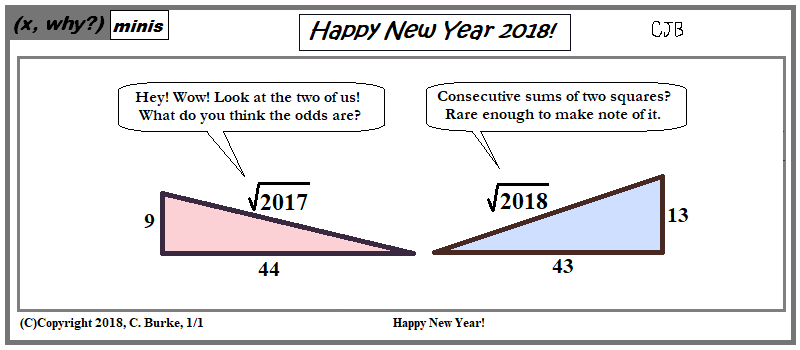Happened 24 years ago, and will happen 24 years from now, but it's not a 24-year cycle.

Here's one list I found on the Internet.

Come back often for more funny math and geeky comics.